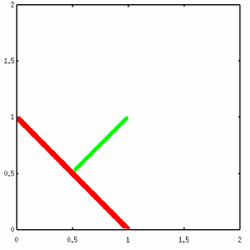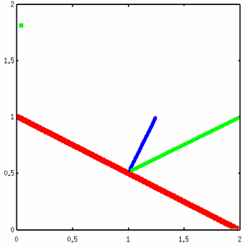Transforming Normals / Tutorial
Basic Technique
When it comes to ray tracing, it's nice to be able to move primitives around in the scene. Otherwise everything is at the origin, and scenes aren't very interesting. So we use affine transformations like scaling, rotation and translation. No problem. However, intersecting with a scaled, rotated, translated primitive can be kind of difficult. The easiest way to deal with it is to keep the primitive at the origin, then apply the inverse of the transformation matrix to the ray instead. If you get a hit, you apply the transformation to move the point of intersection back into world space.

This is all easy. So now you have your point of intersection, you grab the normal from the unit primitive, and use the world transformation to put it into world space too. It's so easy. But it doesn't work. Your scaled primitive is a hole in space. The normals are all wrong.

This problem is common and well-documented. The first place I read about it was in Ray Tracing News volume 0, the article is Abnormal Normals by Eric Haines. See, normals are VECTORS, not points. Your transformation matrices are for points. If you are lucky, most of your transformation matrices will work for vectors, but if you are doing non-uniform scaling you have a problem. And if you have a bad vector class, you're going to have a tough time translating as well.

First we'll deal with a little issue about vectors. If you are doing any real graphics, you are using homogenoeus vectors and matrices. That means 4 dimensions, vectors are [x y z w]. That fourth coordinate is the homogenoeous coordinate, it should be 1 for points and 0 for vectors. The reason will be obvious in a minute. First, realize the difference between points and vectors. Points 'exist' in space (at least theoretically). You can draw a line between two points. Vectors do not. A vector is way of specifying a direction. The vector [1 1 1] means 'go one unit in the positive direction along each axis'. Usually we _represent_ vectors with lines, which is why you might be confused. What we are really drawing is a direction and a magnitude. Hopefully you understand this.

Ok, so why do we have a 'w', or homogeneous coordinate. Couldn't we just leave it off? Well, we could, but then we couldn't have translation matrices that would work for points and vectors. Think about it, a translation matrix looks like this:

 1 0 0 tx 0 1 0 ty 0 0 1 tz 0 0 0 1

Now when you right-multiply by the point [x y z 1], you get [x + tx, y + ty, z + tz, 1]. When you multiply a vector [x y z 0], you get [x y z 0]. Remember, a vector is just a direction. You can't translate a direction, it won't change. The direction [0 0 1 0] is 'one unit up the z axis' no matter where you are in space. We use homogeneous coordinates so we can do translation of points and vectors with the same matrix.

If you are representing points and vectors properly, and you have tried translating, rotating, and uniformly-scaling your objects, you will notice that the 'multiply normal by transformation matrix' scheme actually works. There aren't any problems. But try non-uniform scaling (that means scaling by different amounts in the different axis). Oops. Everything comes crashing down. Now, you could just outlaw non-uniform scaling, but then you can't use spheres to make ellipsoids. And your cones are always going to be at 45 degree angles. This is no good, so a better method is needed.

First a bit of explanation as to why this doesn't work. Look at the graphs below, and pretend that the red line is the line of intersection of the plane x=y with the XY plane. The green line is the normal to this plane at the point [0.5 0.5 0]. The direction of this line is [0.5 0.5 0].

Now we will apply a non-uniform scaling matrix that scales by 2 in the X-axis. The new graph is drawn on the right. The green line is the 'unit' plane normal with the non-uniform scaling matrix applied, it's direction has become [1.0 0.5 0]. This is clearly wrong, the normal should be perpendicular to the plane. In fact, the correct normal is [0.25 0.5 0], which is drawn in blue.So how do we get this normal? The answer is to multiply by the transpose of the inverse of the transformation matrix instead. This is intuitive if you think about it. The transpose of the inverse of a rotation matrix is the same rotation matrix, so rotations are preserved. Translations don't matter because the w coordinate is 0. The only case left is scaling. The inverse of scaling in an axis is 1 divided by the scaling factor. The transpose operation has no effect here, as scaling is all done on the diagonal. Think about stretching a sphere out to be twice as long on the X axis as it is on the Y and Z axis (making an ellipsoid). The surface of the ellipsoid is twice as large, hence the curvature is half what it used to be. So the normal changes half as quickly. Hence when we scale something by 2 in the x axis, we multiply the x component of the normal by one half.

I hope that made sense..

 Questions?Comments? Email rms@unknownroad.com.